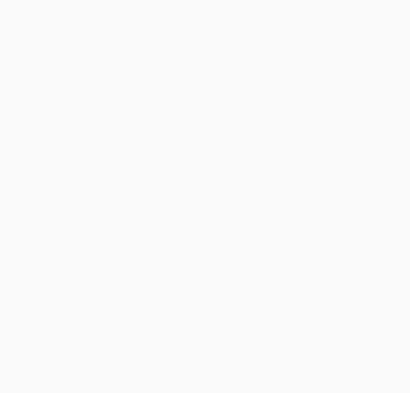# Blitting images onto a tile that is part of a grid in pygame

First of all, t he code which defines and loads the images, can be simplified a lot:

``````il = ['image' + str(i) + '.bmp' for i in range(1,9)]
pygame_image_surfaces = [pygame.image.load(os.path.join(path, name)) for name in imagenames]
``````

The image which is associated to a `Tile` is stored in the instance attribute `self.content` of the `Tile` object. Use this attribute to draw the tile:

``````class Tile:

# [...]

def draw_content(self):
image_rect = self.content.get_rect(center = self.rect.center)
Tile.surface.blit(self.content, image_rect)

def draw(self):
# draw the contents of a tile to its surface.
#  - self: the tile being drawn
self.draw_content()
pygame.draw.rect(Tile.surface, Tile.fg_color, self.rect, Tile.border_width)

def set_content(self, new_content):
#   - self: the tile whose content is being updated
self.content = new_content
``````

Create 1 random list of images. And use this list to set the images for the entire grid of tiles:

``````class Game:

# [...]

def create_grid(self, grid_size):
# Creates a grid of tiles that is grid_size x grid_size in size.

imgnames = ['image' + str(i) + '.bmp' for i in range(1,9)]
image_surfaces = [pygame.image.load(os.path.join(path, name)) for name in imgnames]
image_surfaces = image_surfaces + image_surfaces
random.shuffle(image_surfaces)

self.grid = []
# this for loop creates each row in our grid
for row_num in range(grid_size):
new_row = self.create_row(row_num, grid_size, image_surfaces)
self.grid.append(new_row)

def create_row(self, row_num, size, images):
# Create one row in a grid. Each row contains size Tiles.
# required for calculating the tile's x,y coordinates on screen
#  -  row_num: the nth row of the grid being created
#  -   size  : the number of tiles in the row
# returns the newly created row'

tile_height = self.surface.get_height() // size
tile_width = 3/4*self.surface.get_width() // size
new_row = []
for col_num in range(size):
pos = (row_num * tile_height + 10, col_num * tile_width + 10)
content = images[row_num*size + col_num]
one_tile = Tile(pos, tile_width, tile_height)
one_tile.set_content(content)
new_row.append(one_tile)
return new_row
``````